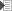# 3.3 Impedance

Impedance is the resistance of a load connected to AC (Alternating Current) instead of DC (Direct Current). Resistance should be seen as impedance at a frequency of 0 Hz (in other words DC). Adding the variable of frequency complicates matters. If a loudspeaker were a simple resistor, its impedance would be a constant throughout its frequency range. But this is not the case. Its impedance changes with different frequencies. Around its resonance frequency the graph shows a high peak, and with higher frequencies, the impedance gradually rises. The specified impedance is really a nominal impedance, measured at 1 kHz. That's exactly why an ohmmeter will never show the real impedance of a cabinet. An ohmmeter measures (DC) resistance. Typically for loudspeakers, their DC resistance is about 80% of their rated impedance.

For a more down-to-earth explanation, see 5.3© Joris van den Heuvel 2001-2009